Mobile QR Code1. (Department of Electronics and Communications Engineering, Kwangwoon University, Seoul 01897, Korea )
2. (College of Information and Communication Engineering, Sungkyunkwan University, Suwon, 16419, Korea )

Ionic screening, electrolyte, field-effect transistor, Monte Carlo method, electrical noise

## I. INTRODUCTION

As the CMOS scaling by Moore’s law has come of an age, the diversification of CMOS technology has been sought in various frontiers in science and engineering. As a notable example, the convergence of nanotechnology and biology is pursued relentlessly, making DNA sequencing possible in affordable costs [1-4]. For further research and development of nanobiosensors, it is imperative to have a clear understanding of signal transduction processes and noise processes in them because the noise sets their sensing limit and the signal needs to be larger than the noise [5-22]. Any change in electric state in the electrolyte region can alter the output of the transducer signal, that is, the drain current of the FET device. To enhance the sensing signal or to control noise properly, we should figure out the electric transfer characteristics quantifying how the electric signal is transferred from the signal source to the destination.

We conduct a particle simulation to understand the signal transduction processes in noisy electrolyte environment. There have been many previous studies on the noise in electrolyte-insulator-semiconductor field-effect transistors (EISFETs) [6-8,10,18,19]. Many models and experimental results have been reported [13-17]. However, the previous studies have been based on the continuum models [8,10,16-18]. It is not easy to find particle simulation studies which focus on the electrical transfer characteristics from the oscillating charged objects in the electrolyte to the drain current in high frequency as well as in low frequency regions.

Nanobiosensors have a fundamental sensing limit due to the Debye-Hückel screening effect when the nanobiosensors work in a physiologically relevant electrolyte [23-28]. To overcome this deleterious effect, one approach was presented to use the high-frequency signal to overcome the screening effect .

In this paper, by using the Monte Carlo particle simulation, we investigate how the screening length changes as the oscillation frequency of the charged molecule or object increases. Also, the dependency of the ionic screening effect on the distance of the target molecule from the electrolyte-insulator interface is studied and a simple model is presented to understand the frequency dependence of the screening effect.

This paper consists of as follows: Section II explains the simulation method. Section III shows the simulation results of the electric transfer coefficient and a capacitance model is given. Conclusions follow in Sec. IV.

## II. THE SIMULATION METHOD

We have exploited a particle simulation method to monitor the electrical processes of biosensor devices, especially in the electrolyte-insulator-semiconductor system. We already conducted analyses of noise characteristics induced by ion motion in the electrolyte using the simulation method, where the usefulness of the simulation method is best shown in Ref. 19. The followings are a brief description of the particle simulation method. Fig. 1 shows a schematic diagram of the simulation domain which includes an FET channel, a dielectric, and an electrolyte region proximate to the dielectric interface. Its horizontal plane ($x-y$) is set to be 0.2${\times}$0.2 μm$^{2}$, comparable to the size of nanobiosensor devices, considering a compromise between simulation time and accuracy. The domain heights ($z$-direction) used in the simulations are 0.3${-}$0.5 μm which are sufficiently larger than the Debye lengths of the electrolyte. The domain may contain dielectric spheres to model molecules or objects in the electrolyte region which have some amount of charges at their center points, located apart from the electrolyte-dielectric interface by a certain distance.

The simulator computes motion of ion particles in the electrolyte region. Basically, ion particles move randomly in the electrolyte by thermal energy and scattering processes, called the Brownian motion. Though the velocity distribution and the mean free path of ion particles are not clearly known, we can substitute the effective traveling velocity which is derived from measured diffusivity values, depending on the average flight length. While the real mean free path of scattering ions may be comparable to the distance between adjacent water molecules which can be obtained approximately from the sphere packing model, we increase, however, the average flight length by three times to accelerate the simulation. We also use a physical scattering model such that an ion collides with a sphere-shaped water molecule, automatically conserving the average kinetic energy of ion particles. Monte Carlo method is applied to pick the flight length and the collision angle of a scattering process, which enables to simulate noise caused by ion’s random motion.

Two kinds of forces exerted on ion particles are calculated in this simulation: Coulomb force between charged particles and the Lennard-Jones force between proximate particles [26,27]. Because an ion is not a free particle but in strong association with water molecules, very little portion of Coulomb force contributes to the traveling velocity of the ions. We have set up the magnitude of Coulomb force to cause the drift velocity so that the ion concentration should follow the Poisson-Boltzmann distribution. The background water is treated as dielectric material with relative permittivity of 79.

The mean time step is around 0.5 ns which is much longer than those used in the semiconductor MC simulations. Diffusivities of ions in the electrolyte are of the order of 10$^{-5}$ cm$^{2}$/s, while electron in silicon has the diffusivity of ~10$^{1}$ cm$^{2}$/s . The long simulation time step which is possible due to such a low mobility enables one to simulate the low frequency characteristics down to ~kHz. Since the number of simulated particles is relatively small, it is time-efficient to calculate the electric field at each ion’s position by the Coulomb’s law, rather than to solve the Poisson equation over the entire domain with mesh structure.

The bottom plane of the domain is assigned as the FET channel region which is treated as an electrode in the simulation. The FET channel charges are increased or decreased so that the potential drop between the top and the bottom planes of the domain should be equal to the bias voltage, $V$$_{SG}, between the FET source and the reference electrode which is placed outside of the simulation domain, holding the electrostatic potential of the bulk electrolyte region. The boundary surfaces should handle two kinds of physical processes. As common to any type of electrical simulations, the electric (E)-field or potential condition at the boundary surface must be given. At the bottom plane, the electric potential is set to zero. Fig. 1. (a) Schematic view of simulation domain comprised of the electrolyte region, the thin insulator layer and the FET channel plane. The electrolyte region contains ions and a charged target object at position {d} apart from the electrolyte-insulator interface. The shaded region at the upper part is used for ‘diffusion-rate monitoring’, (b) Simulation domain as part of the EISFET sensor system.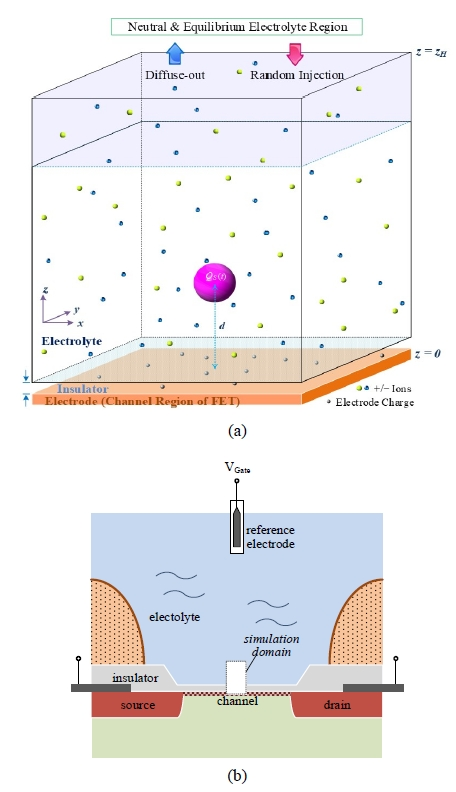Vertical E-field value, calculated from the domain net charge and Gauss’ law, is applied to the top boundary surface. For the boundaries in the horizontal directions, we employ the periodic boundary condition, repeating the domain in the x- and y- directions like a checkerboard pattern [30-32]. The other boundary process is about entrance and exit of simulated ion particles. Ion particles are injected into the domain with a probability in the top boundary plane as if the domain is contiguous to the electrolyte of the basic ion concentration in the z-direction. In simulation time steps, ions inside the domain may go out across the upper z-boundary by the Brownian motion, i.e. the diffusion process. Fig. 2. Simulated anion and cation concentration in electrolyte surrounding a positively charged sphere along the distance from the sphere surface, showing that the simulation results fit very well to the Poisson-Boltzmann distribution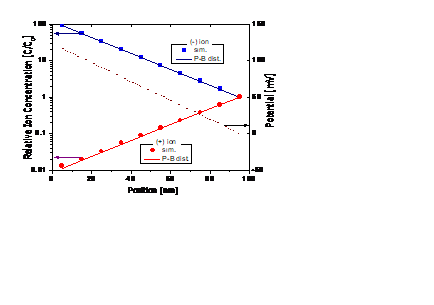A diffusion rate at which the inside ions go out is calculated taking the ion concentration of the ‘diffusion-rate monitoring’ region into account. Events that an ion is injected into the domain through the upper z-boundary take places randomly but at the diffusion-rate. The initial number of particles in the domain is deduced from the ion concentration of the hypothetical electrolyte. The simulated ion particles consist of equal number of monovalent anions and cations. We employ 5.23{\times}10^{16} cm^{{-}3} (0.045 mM) for basic ion concentration in our work, equivalent to 326 anion and cation particles in this domain, respectively. However, the ion particle number of each polarity fluctuates and drifts, depending on the charge amount in the dielectric sphere. From an electrical point of view, the overall EIS system can be considered as a capacitor, where the reference electrode and the FET channel correspond to its two terminals. Our simulation implements biasing of the gate voltage in a similar way to biasing of a capacitor: Current flows into or out of the terminal if the internal Coulomb voltage between the two terminals (V$$_{gs,int}$) is not equal to the applied external voltage ($V$$_{gs,ext}). Charges are added to or subtracted from the FET channel at each simulation step, to balance V$$_{gs,int}$ and $V$$_{gs,ext}. The result of the simulation yields the amount of channel charge at each time step, which if the FET is in the deep linear mode, is proportional to the drain current. Before starting the main simulations, we simulate the ion concentration profiles around a charged material to check the validity of simulation. A dielectric sphere of radius of 10 nm containing +30 q’s at its center point is deposited in the electrolyte region where ions are uniformly distributed as an initial state. Fig. 2 shows the ion concentration profiles along the distance from the dielectric sphere surface after settling into an equilibrium state, time-averaged over 20 μs after 10 μs past the start. They are in good agreement with the Poisson-Boltzmann distribution having a Debye length of 43 nm that is calculated with 5.23{\times}10^{16} cm^{{-}3} (0.045 mM) monovalent ion concentration. ## III. ELECTRICAL TRANSFER SIMULATION RESULTS Charged molecules or objects bound with bio-receptors near the surface are expected to generate electric fields and to induce the field effect to the transistor channel area. However, it is widely accepted that the electric field from the charged target is screened by surrounding electrolyte ions and does not reach the FET surface if the charged material is located farther from the surface by more than the Debye length, {\lambda}$$_{D}$, and this phenomenon is called the screening effect [4,17,26,28]. Many articles on the affinity-based FET sensors are based on a simple assumption that the charged materials located beyond ${\lambda}$$_{D} from the channel surface do not contribute to any field effect while nearer ones induce the full image charge on the FET channel, which is too rough to describe the real phenomena [17,28]. It is not easy to find the literature which deal with the quantitative assessment of how much image charge the charged molecule/object induces on the FET channel as the charged object’s distance from the surface changes. The particle simulation method can be used to quantitively investigate the screening effect. To quantify this effect, we introduce a figure of merit, an electric transfer coefficient (ETC), defined as the ratio of the image charge induced on the FET channel (Q$$_{C}$, channel charge) to the charge of target material in the electrolyte ($Q$$_{S}, source charge). We also call various phenomena related to the ETC features by a new term, electric transfer characteristics. The charge distribution on the FET channel surface induced by a charged sphere is simulated first by using the particle simulation method. An invariant amount of charge (+30q) is assigned to the center of a sphere, which is modeled as a target material in the electrolyte. Fig. 3. Amounts of image charge induced on the FET channel depending on the distance between the center point of the charged sphere and the electrolyte-insulator interface. The object sphere has a constant amount of charge (DC). The blue line indicates the simple geometric portion of shielding charge covered by the FET channel region as illustrated in Fig. 4.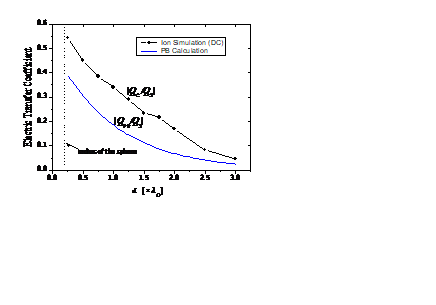The sphere has a radius of 10 nm and its center point is located distance d apart from the electrolyte-insulator interface, as illustrated in Fig. 1. According to Gauss’ law, a lump charge at the center point is electrically equivalent to the same amount of uniformly distributed charge on the sphere surface which conforms to actual charged materials. From the ion concentration (5.23{\times}10^{16 }cm^{{-}3}) of the electrolyte, the Debye length ({\lambda}$$_{D}$) is calculated to be 43 nm. The dielectric sphere with a radius of 10 nm is placed at locations $d$ = 0.25${-}$2.0${\lambda}$$_{D} from the electrolyte-insulator interface. The simulation results which the particle simulations bring about fluctuate over time, reproducing noise induced by ion’s thermal motion. The stable DC values of the FET channel charge can be obtained by averaging the simulation output over an adequate simulation time. Fig. 3 plots the DC ETC values as the distance d varies. If the spherical object contains an amount of the source charge, Q$$_{S}$, it attracts the same amount of charge opposite in polarity, ${-}$$Q$$_{S}$ (compensation charge) to its nearby space. The compensation charge is dispersed following the Poisson-Boltzmann (PB) equation in the homogeneous electrolyte surroundings [26,27,33]. However, the existence of the FET channel surface distorts the electric field and the charge distribution. Fig. 3 shows that up to 60 \% of the compensation charge lies on the FET channel surface when the charged sphere is adjacent to the interface. Considering that the radius of the sphere is approximately a half ${\lambda}$$_{D}, a much greater portion of charge appears as the image charge on the FET channel than expected from the simple geometric portion as plotted in the blue line in Fig. 3. Here, the concentration of the shielding charge around the sphere is assumed to follow the PB distribution regardless of the existence of the FET channel as if an electrolyte environment extends infinitely. An attached graph in Fig. 4 schematically draws the charge concentration along r (distance from the sphere surface). We calculate Q$$_{PB}$, the amount of charge occupying the space below the electrolyte-insulator interface through a numerical method under the assumption of the infinite electrolyte region. The simulated ETC is 0.34 at 1.0${\lambda}$$_{D} distance and not very low until around 2.0{\lambda}$$_{D}$ distance, while a conventional rule of thumb ignores the field effect by charges located farther than ${\lambda}$$_{D} from the interface. The Q$$_{C}$’s of the particle simulations are also considerably higher than the simple $Q$$_{PB}’s because the potential profile in the electrolyte region is altered by the existence of the FET channel. If the amount of the source charge is time-varying, the image charge on the FET channel responds to the source charge. This response is dependent on the frequency of the source charge due to ion behaviors in the electrolyte. The particle simulation method provides an appropriate tool for investigating the frequency-dependent electric transfer characteristics. The Q$$_{S}$($t$) (=$q$·$N$$_S(t), source signal) is now set to be a sinusoidal wave with a single frequency. Then, the signal of the same frequency component (transferred signal) is extracted from the simulated Q$$_{C}$($t$) (= ${-}$$q·N$$_C$($t$)), where the minus sign indicates the polarity of the charge opposite to the $Q$$_{S}. The ETC can be redefined as the ratio of the Fourier coefficient of the transferred signal to that of the source signal at the same frequency. Fig. 5 is an instance of the simulated results where N$$_{S}$($t$)=30∙sin(2πx16000$t$), showing how to analyze the frequency response. The value on the y-axis indicates the squared magnitude of the Fourier transform of $N$$_{S}(t) and N$$_{C}$($t$), corresponding to the signal power per Hz. The blue line verifies that the source signal is a nearly-single tone at 16 kHz. Through the particle simulations, the number of charges induced on the FET channel is obtained as $N$$_{C}(t). The signal level of N$$_{C}$($t$) plotted in the red line consists of the single-tone signal in response to $N$$_{S}(t) and the Lorentzian-shaped noise spectrum caused by motion of electrolyte ions. As investigated in our previous work , the thermo-Coulombic motion of electrolyte ions induces the Lorentzian-type noise in the FET drain current by modulating the carrier number on the FET channel region. Fig. 4. Amount of shielding charge (Q$$_{PB}$) occupying the geometric space below the electrolyte-insulator interface. The shielding charge is assumed to follow the PB distribution regardless of the existence of the FET channel in this calculation.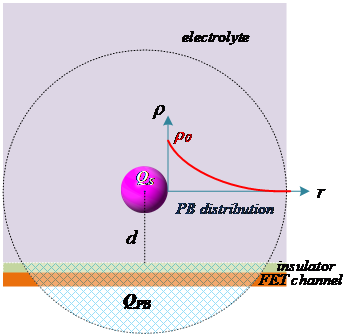The graphs in Fig. 5 are drawn in the same way as the power spectral density except that the channel electron density, $N$$_{C}(t) is used instead of the drain current. Since N$$_{C}$($t$) is directly proportional to the drain current when the details in the device are specified, the red line in the figure can be regarded to represent the power spectral density of the drain current.

To obtain accurate ETC values, the long simulation time is necessary in such a way that the single-tone should exceed far beyond the background Lorentzian noise level in magnitude. As the simulation time increases, the power of the single-tone response grows faster than the background noise. The simulation time must be longer than multiple folds of the period of the sinusoidal signal, especially for the low frequencies.

As previously discussed, the ETC is clearly dependent on the distance between the dielectric sphere and the FET surface. We simulate the frequency dependency of the ETC from 250 Hz to 30 MHz. Since the simulations at the lower frequencies require too long time for simulation, we confirm the consistency of the simulation results in the very low frequencies by comparing them with the DC values. To integrate the signal at least for two periods, the simulation times are set as 8 ms for the low frequencies. For higher frequencies than 2 MHz, the simulation times are shortened to 2 ms. In Fig. 6(a), the magnitudes of ETC agree well with the DC values in the low frequency range until around 5${-}$10 kHz, showing the dependency on $d$, too.

Fig. 5. An instance of the ion simulation results showing how to analyze the responses in the frequency domain. The power spectral density profile of $N$$_{C}(t) (red line) in response to the signal source, N$$_{S}$($t$) (blue line) is obtained through the ion simulation.However, they undergo transitions from the low values to the high values as the frequency increases. The transition begins at around 10 kHz and finishes at 100 kHz. The frequency where the transition begins coincides with the corner frequency of the background Lorentzian-shaped noise, implying that the transition frequency is related to the RC value of the bulk electrolyte . While as the ETC values at the low frequencies are strongly dependent on the distance between the dielectric sphere and the FET channel, the dependency on the distance becomes weaker as the frequency goes beyond the transition frequency.

Fig. 6(b) shows the phases of the ETC’s. Zero phase means that the response of the $N$$_{C}(t) to the N$$_{S}$($t$) signal is instantaneous compared to the signal period. The higher phase means a lagging response. The phase values are fluctuating in the low frequency region where the background noise level is high. Nonetheless, it is not difficult to grasp that the frequency range where the phase shifts agrees with the transition range of the magnitude of ETC in Fig. 6(a). We also find that the phase shifts more as the distance $d$ increases. This may give a clue to establishing a more accurate electric model of the electrolyte.

In the high frequency region, the signal is transmitted mainly via the capacitance components of the electrolyte region. Seeing that the ETC values decrease too as the distance $d$ increases in Fig. 6(a), we presume that the ETC magnitude level in the high frequency region is determined by geometric factors. Fig. 7 illustrates the simulation domain with a capacitance model and its boundary condition on the top $z$-plane ($z$=$z$$_{H}) conceptually. Fig. 6. Simulated frequency characteristics of the ETC value depending on the distance between the charged object and the electrolyte-insulator interface (a) in the magnitude, (b) in the phase.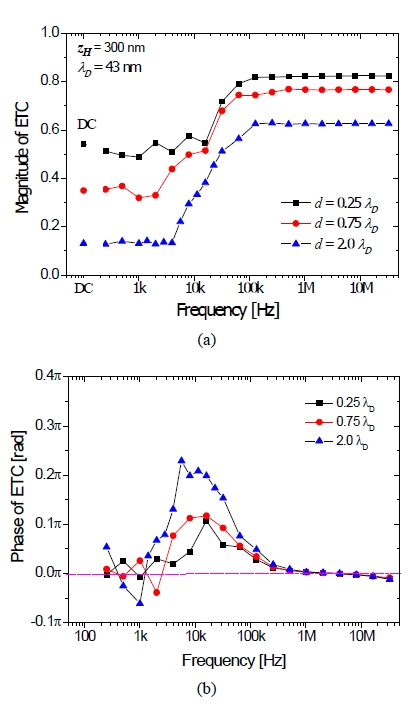In case of DC, the electric potential at a point on the top z-plane is a function of the net charge inside the simulation domain, being derived by using a Gaussian surface on the top z-plane. In our simulation, the static boundary condition is assumed on the top z-plane surface (z=z$$_{H}$), so that the electric potential, i.e. the voltage drop applied across the electrolyte region outside the domain is an instantaneous function of the net charge amount inside the domain, given by Eq. (1)which is derived by the Poisson-Boltzmann equation.

where $V$$_{th} is the thermal voltage, Q is 1.6{\times}10^{{-}19} C, the unit charge, {\varepsilon}$$_{w}$ is the electrical permittivity of the water, $C$$_{0} is the basic ion concentration, Q$$_{IN}$ is the net charge inside the domain and $A$ is the surface area of the top $z$-plane. This is not an accurate modeling of actual phenomena especially at high frequencies because it neglects the time transient effect of ion redistribution outside the domain. Although it introduces an error factor, it is inevitable to use such a simple DC boundary condition under the limitation of simulation domain. This boundary condition can be modeled as a capacitor from Eq. (1)and the capacitance value is calculated as in Eq. (2).

The $C$$_{out} per unit area presented in Eq. (2) is about 10 times larger than C$$_{elb}$ which is the capacitance between the dielectric sphere and the top $z$-plane. Since the top $z$-plane boundary surface almost acts like an AC ground in the high frequency region with our boundary condition, the ETC is determined only by the geometrical factor in this region. To verify the above elucidation of ETC dependency on the distance in the high frequency region, another set of simulations are conducted. If the simulation results are affected by extension of the domain in the $z$-direction, we can draw a conclusion that the imperfect boundary condition is responsible for it.

Two more cases about the top $z$-plane positions ($z$$_{H} = 300, 400 nm) in addition to the previous case (z$$_{H}$ = 200~nm) are simulated with the same simulation setups ($d$ = 2.0${\lambda}$$_{D}). From the simulation results shown in Fig. 8, it seems apparent that the position of domain boundary has a direct influence on the ETC value in the high frequency range. The ETC in the high frequency region is reduced as z$$_{H}$ is increased. It is properly explained through the model in Fig. 7. As the top $z$-plane boundary becomes farther from the sphere, $C$$_{elb} decreases and thus the source signal, Q$$_{S}$($t$) is more tightly coupled to the FET channel plane, leading to higher ETC values. This model explains why the high frequency ETC’s have different values depending on the distance $d$. The coupling ratio of $C$$_{elf}$$C$$_{elb} increases as the charged sphere becomes closer to the FET interface. In the case of real sensing system, there is not any kind of boundary within hundred folds of {\lambda}$$_{D}$ in the $z$-direction, implying that the high frequency ETC approaches unity independently of the distance $d$.

Fig. 7. A capacitance model of the simulation domain and the top $z$-plane boundary, explaining that the position of top $z$-plane boundary may affect the ETC values in the simulations.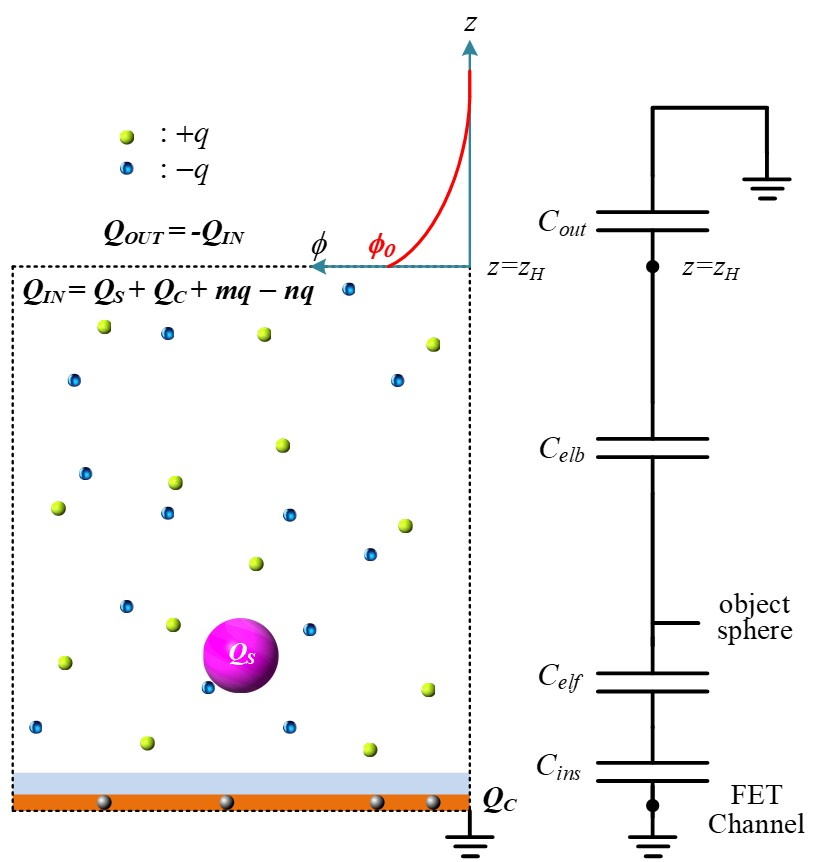Fig. 8. Simulated frequency characteristics of the ETC magnitude with altered heights ($z$$_{H}) of the simulation domain.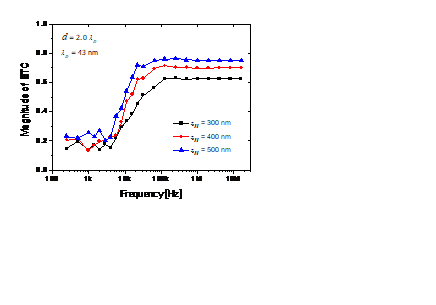Results of our particle simulation suggest two meaningful points about the screening effect of the electrolyte in the biosensor system. For target materials with the DC or low frequency charge, though the charge signal is shielded by the screening effect, a considerable portion of the charge is induced on the FET channel even in case d > {\lambda}$$_{D}$. If the charge in the target object is varying in high frequency, the whole charge appears as the image charge on the channel, being free from the screening effect. It is hard to imagine that a target molecule for bio-sensing has a time-varying charge with high frequency. However, the target object can possibly have a dipole oscillating at high frequency by the nonlinear mixing between the alternating current excitation field and the molecular dipole field . In addition, the oscillation of the position of the charged object also might give rise to the similar effect from a standpoint of the field effect on the FET channel.

We expect that this particle simulation platform can be extended to investigate the low-frequency noise related to the kinetic properties at the electrolyte-insulator interface , and charge noise in organic electrochemical transistors [20,22].

## V. CONCLUSIONS

We developed the particle simulator to study the signal transfer from the charged target to the drain current when the target charge oscillates. We studied how the Debye-Hückel screening effect in the electrolyte can be overcome as the target charge’s oscillation frequency increases. A simple capacitance model was presented to understand that the high frequency oscillation of the target charge can bypass the screening effect. It is expected that with this AC method, a nanobiosensor can be developed to have a higher sensitivity than those of nanobiosensors operating in DC or low-frequency regions.

### ACKNOWLEDGMENTS

This work was supported by the Research Grant of Kwangwoon University in 2019.

### REFERENCES

1
Bergveld P., 2002, Thirty years of ISFETOLOGY: What happened in the past 30 years and what may happen in the next 30 years, Sens. Actuators B, Vol. 88, pp. 1-202
Stern E., Klemic J. F., Routenberg D. A., Wyrembak P. N., Turner-Evans D. B., Hamilton A. D., LaVan D. A., Fahmy T. M., Reed M. A., 2007, Label-free immunodetection with CMOS-compatible semiconducting nanowires, Nature, Vol. 445, pp. 519-5223
Rothberg J. M., 2011, An integrated semiconductor device enabling non-optical genome sequencing, Nature, Vol. 475, pp. 348-3524
Alam M. A., 2013, Principles of Electronic Nanobio-sensors, www.nanohub.org5
Nemirovsky C. G., Jakobson Y., 1999, 1/f noise in ion sensitive field effect transistors from subthreshold to saturation, IEEE Trans. Electron. Dev., Vol. 46, pp. 259-2616
Hassibi A., Navid R., Dutton R. W., Lee T. H., 2004, Comprehensive study of noise processes in electrode electrolyte interfaces, J. Appl. Phys., Vol. 96, pp. 1074-10827
Hassibi A., Zahedi S., Navid R., Dutton R. W., Lee T. H., 2005, Biological shot-noise and quantum-limited signal-to-noise ratio in affinity-based biosensors, J. Appl. Phys., Vol. 97, pp. 084701-1-108
Landheer D., Aers G., McKinnon W. R., Deen M. J., Ranuarez J. C., 2005, Model for the field effect from layers of biological macromolecules on the gates of metal-oxide-semiconductor transistors, J. Appl. Phys., Vol. 98, pp. 044701-1-159
Fromherz M., Voelker P., 2006, Nyquist Noise of Cell Adhesion Detected in a Neuron-Silicon Transistor, Phys. Rev. Lett., Vol. 96, pp. 228102-1-410
Deen M. J., Shinwari M. W., Ranuárez J. C., Landheer D., 2006, Noise considerations in field-effect biosensors, J. Appl. Phys., Vol. 100, pp. 074703-1-811
Hassibi A., Vikalo H., Hajimiri A., 2007, On noise processes and limits of performance in biosensors, J. Appl. Phys., Vol. 102, pp. 014909-1-1212
Tersoff J., 2007, Low-Frequency Noise in Nanoscale Ballistic Transistors, Nano Lett., Vol. 7, pp. 194-19813
Gao X. P. A., Zheng G., Lieber C. M., 2010, Subthreshold Regime has the Optimal Sensitivity for Nanowire FET Biosensors, Nano Lett., Vol. 10, pp. 547-55214
Zheng G., Gao X. P. A., Lieber C. M., 2010, Frequency domain detection of biomolecules using silicon nanowire biosensors, Nano Lett., Vol. 10, pp. 3179-318315
Heller I., Chatoor S., Männik J., Zevenbergen M. A. G., Oostinga J. B., Morpurgo A. F., Dekker C., Lemay S. G., 2010, Charge Noise in Graphene Transistors, Nano Lett., Vol. 10, pp. 1563-156716
Go J., Alam M. A., 2013, The future scalability of pH-based genome sequencers: A theoretical perspective, J. Appl. Phys., Vol. 114, pp. 164311-1-10-17
Georgakopoulou K., Birbas A., Spathis C., 2015, Modeling of fluctuation processes on the biochemically sensorial surface of silicon nanowire field-effect transistors, J. Appl. Phys., Vol. 117, pp. 104505-1–818
Park C. H., Chung I.-Y., 2016, Modeling of Electrolyte Thermal Noise in Electrolyte-Oxide-Semiconductor Field-Effect Transistors, J. Semicon. Tech. & Sci., Vol. 16, pp. 106-11119
Chung I.-Y., Lee J., Seo M., Park C. H., 2016, Particle simulation of electrolytic ion motions for noise in electrolyte–insulator–semiconductor field-effect transistors, Jap. J. Appl. Phys., Vol. 55, pp. 127001-1-720
Crosser M. S., Brown M. A., McEuen P. L., Minot E. D., 2015, Determination of the Thermal Noise Limit of Graphene Biotransistors, Nano Lett., Vol. 15, pp. 5404-540721
Zhang D., Solomon P., Zhang S.-L., Zhang Z., 2017, Correlation of Low-Frequency Noise to the Dynamic Properties of the Sensing Surface in Electrolytes, ACS Sens., Vol. 2, pp. 1160-116622
Stoop R. L., Thodkar K., Sessolo M., Bolink H. J., Schönenberger C., Calame M., 2017, Charge Noise in Organic Electrochemical Transistors, Phys. Rev. Appl., Vol. 7, pp. 014009-1-823
Bockris J. O’M., Reddy A. K. N., Gamboa-Aldeco M., 2000, Modern Electrochemistry: Fundamentals of Electrodics, Vol. 2A (Kluwer Academic, 2000) , No. 2nd ed24
Gileadi E., 1993, Electrode Kinetics for Chemists, Chemical Engineers, and Material Scientist, Wiley-VCH25
Bard A. J., Faulkner L. J., 2001, Electrochemical Methods: Fundamentals and Applications, Wiley, No. 2nd ed.26
Dill Ken A., Bromberg Sarina, 2011, Molecular Driving Forces, 2nd ed.28
Kulkarni G. S., Zhong Z., 2012, Detection beyond the Debye Screening Length in a High-Frequency Nanoelectronic Biosensor, Nano Lett., Vol. 12, pp. 719-72329
Cussler E. L., 2009, Diffusion, Cambridge Univ. Press, Cambridge, No. 3rd ed., pp. 126-13030
Rapaport D. C., 2004, The Art of Molecular Dynamics Simulation, Cambridge Univ. Press, Cambridge, No. 2nd ed., pp. 15-20.31
Smit D. Frenkel and B., 2002, Understanding Molecular Simulation, Academic Press, San Diego, No. 2nd ed., pp. 3432
Hockney R. W., Eastwood J. W., 1988, Computer Simulaiton Using Particles33
Vlachy V., 1999, Ionic effects beyond Poisson-Boltzmann theory, Annu. Rev. Phys. Chem., Vol. 50, pp. 145-165## Author

In-Young Chung received the B.S., M.S., and Ph.D. degrees in elec-tronics engineering from Seoul National University, Seoul, Korea, in 1994, 1996, and 2000, respectively.

He worked at Samsung Electronics from 2000 to 2004, where he designed DRAM circuits.

He joined Gyeongsang National University as a faculty member in 2004.

Since 2008, he has been with Kwangwoon University, Seoul, where he is currently a Full Professor with the Department of Electronics and Communications Engineering.

He was a visiting scholar at University of California at Santa Barbara in 2014.

His current research interests include the simulation and modeling of nanodevices and biosensors.

Munkyo Seo received his B.S. and M.S. degrees in electronics engi-neering from Seoul National University, Korea, in 1994 and 1996, respectively, and Ph.D. degree in electrical engineering from the University of California, Santa Barbara, CA USA, in 2007.

From 1997 to 2002, he was with LG Electronics Inc., designing RF subsystems for wireless communication.

In 2009, he joined Teledyne Scientific Company, Thousand Oaks, CA USA, where he worked on the design of millimeter-wave/terahertz circuits and high-speed mixed-signal circuits. Since 2013, he has been with the College of Information and Communication Engineering, Sungkyunkwan University, Korea, where he is now an Associate Professor.

His research interests include design of millimeter-wave/terahertz circuits and systems for communication, radar and sensors.

Chan Hyeong Park received the B.S., M.S., and Ph.D. degrees in electronics engineering from Seoul National University, Seoul, Korea, in 1992, 1994, and 2000, respectively.

From 2000 to 2003, and from 2009 to 2012 he was a Visiting Scientist and a Visiting Professor, respectively with the Research Laboratory of Electronics, Massachusetts Institute of Technology (MIT), Cambridge.

Since 2003, he has been with Kwangwoon University, Seoul, where he is currently a Full Professor with the Department of Electronics and Communications Engineering, His current research interests include the modeling of noise processes in semiconductor devices and biosensors.